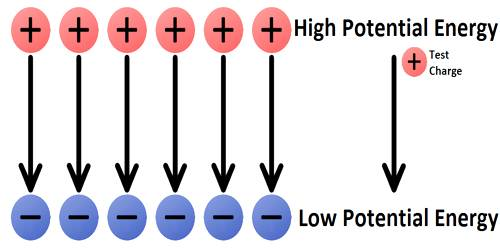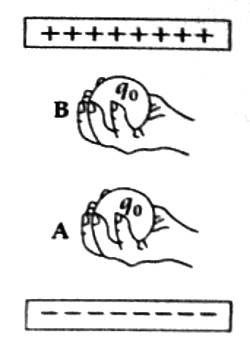# Electric Potential Energy

Electric potential energy

Electric potential energy is the energy a charge has due to its position relative to other charges. If you take a ball with mass m and raise it to any height, you are giving it gravitational potential energy.

Let a test charge q0 be at rest between two oppositely charged plates [Figure]. Since the plates are charged, the test charge will be attracted by the lower plate. Let the test charge be kept to rest at the position. A by applying some external force (say by hand). Now, let the charge be taken from position A to position B against the attractive force by applying some external force. The external force does work in taking the charge from A to B. According to work-energy principle, the work done is equal to the change in total energy.Now, at positions A and B the body, is at rest, so there will be no change in kinetic energy; only change in potential energy will take place. Since the phenomenon is electrical, so the associated potential energy is called electrical potential energy. Now according to work-energy theorem work done WAB is equal to charge in electrical potential energy. That is-

WAB = EB – EA

Here EB and EA are the electric potential energy at point B and A respectively. Since electric force is a conservative force, so work done WAB in taking the test charge from A to B is independent of the path i.e., WAB will be same for any path. As the electric force resisting the motion of the test charge is dependent on the amount of charge [since F = Eq0], so work done in taking the test charge from points A to B depends on the amount of charge. Hence, it is convenient to consider work done for unit charge. Hence work done per unit charge.

WAB/q0 = (EB – EA) / q0 = (EB / q0) – (EA / q0)

This potential energy per unit charge is called electric potential or simply potential.

WAB/q0 = VB – VA

Here, VB and VA are respectively the potentials at point B and A.